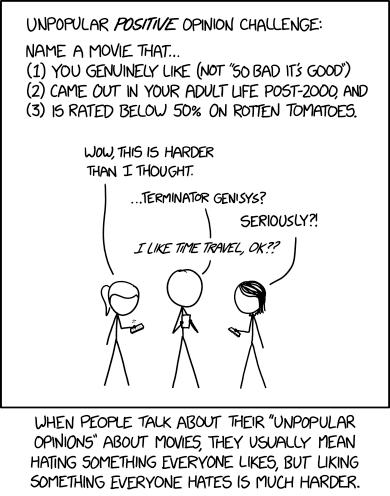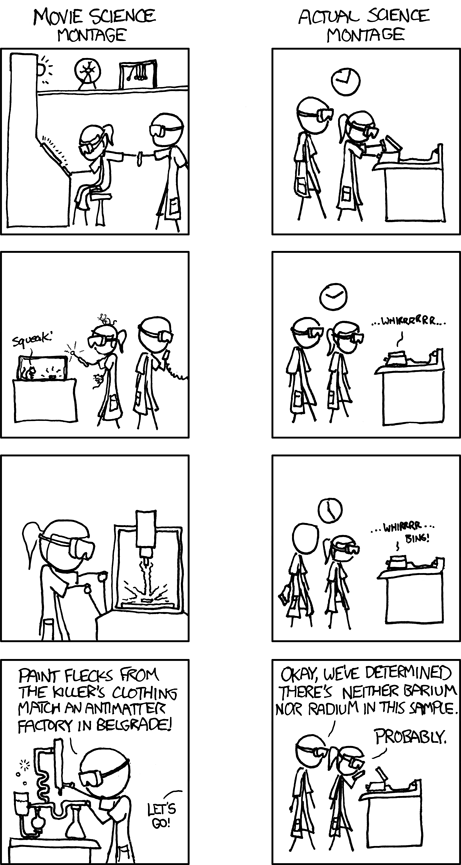## Unpopular positive opinion challengeChallenge accepted. I got three, though none are below 40%.

## The Yellow Birds (2018), 45% on RT

The market isn’t so hot for Iraq war movies. And it’s nearly impossible to adapt such an introspective novel into film. I still respect the effort and its outcome, even if all references to the normal distribution got left out of it.

I spent a lot of time trying to identify the exact point at which I noticed a change in Murph, somehow thinking that if I could figure out where he had begun to slide down the curve of the bell that I could do something about it. But these are subtle shifts, and trying to distinguish them is like trying to measure the degrees of gray when evening comes. It’s impossible to identify the cause of anything, and I began to see the war as a big joke, for how cruel it was, for how desperately I wanted to measure the particulars of Murph’s new, strange behavior and trace it back to one moment, to one cause, to one thing I would not be guilty of. And I realized very suddenly one afternoon while throwing rocks into a bucket in a daze that the joke was in fact on me. Because how can you measure deviation if you don’t know the mean? There was no center in the world. The curves of all our bells were cracked.

(From The Yellow Birds by Kevin Powers)

## Hearts in Atlantis (2001), 49% on RT

Two actors with (essentially) the same first name and over 50 years of age difference (Anthony Hopkins 1937-, Anton Yelchin 1989-2016) make this Stephen King adaptation well worth watching.

He made another circuit of his room, working the tingles out of his legs, feeling like a prisoner pacing his cell. The door had no lock on it—no more than his mom’s did—but he felt like a jailbird just the same. He was afraid to go out. She hadn’t called him for supper, and although he was hungry—a little, anyway—he was afraid to go out. He was afraid of how he might find her… or of not finding her at all. Suppose she had decided she’d finally had enough of Bobby-O, stupid lying little Bobby-O, his father’s son? Even if she was here, and seemingly back to normal… was there even such a thing as normal? People had terrible things behind their faces sometimes. He knew that now.

(From Low Men in Yellow Coats by Stephen King)

## Maze Runner: The Death Cure (2018), 43% on RT

Sure, it’s not as good as the first film in the series (which does not qualify for the challenge, scoring 65% on RT), but a major improvement on the mindless zombie chases of the second part. I like to think of it as a parable illustrating ethical issues in public health… allowing for the customary movie-science vs actual-science differences.## “Full dimensional sets without given patterns” by Péter Maga

The footnote says that the paper is a part of the authors’ Master’s thesis at Eötvös Loránd University. Well, they write neat Master’s theses over in Budapest. The paper is available online as a preprint and in final version (by subscription). I rewrote my Zentralblatt review into something more resembling a blog post.

In analogy with additive number theory, I am going to define additive geometric measure theory as the search for arithmetic/geometric patterns in sufficiently large subsets of$\mathbb R^n$. Given two sets$A$ and$P$ in$\mathbb R^n$, one says that$A$ contains$P$ as a pattern if there exists a similarity$\phi\colon \mathbb R^n\to\mathbb R^n$ such that$\phi(P)\subset A$. (A similarity is a map that multiplies all distances by the same nonzero factor.)

It is not hard to see (via Lebesgue density theorem) that a set of positive measure contains every finite set as a pattern. Erdős conjectured that finiteness is essential here: for any infinite pattern$P$ there is a positive-measure set$A$ which avoids it. This conjecture of Erdős is still open. The paper investigates the opposite direction: the patterns are finite, but the sets which contain (or avoid them) are smaller. There is nothing interesting about 1- and 2-point patterns, so the first case of interest is a 3-point pattern.

Tamás Keleti proved that for every three-point subset$P\subset \mathbb R$ there exists a compact set$A\subset \mathbb R$ of Hausdorff dimension$1$ which avoids$P$ as a pattern (actually, his sets can avoid countably many such patterns at once). One of the results of the present article achieves the same goal in$\mathbb R^2$: for any three-point subset$P\subset \mathbb R^2$ there exists a compact set$A\subset \mathbb R^2$ of full Hausdorff dimension (that is,$2$) which does not contain$P$ as a pattern. The higher dimensional version remains open.

The two dimensional case is special because the triangle-avoiding property can be related to the arithmetics of complex numbers. Given a set$A\subset \mathbb C$, let$\mathcal T(A)=\left\{\frac{z-x}{y-x} \colon x,y,z\in A, x\ne y\right\}$ be its divided difference set (compare to the usual difference set$\mathcal D(A)=\left\{{y-x} \colon x,y\in A\right\}$). Up to a similarity transformation, every nondegenerate triangle can be encoded by a complex number$\zeta\ne 0$. Actually,$\zeta$ is not unique: the numbers$\zeta, \zeta^{-1}, 1-\zeta, (1-\zeta)^{-1}, 1-\zeta^{-1}, (1-\zeta^{-1})^{-1}$ represent the same triangular pattern. According to the old book Automorphic Forms by Lester Ford, this incarnation of$D_3$ is called the group of anharmonic ratios, or maybe just the anharmonic group. I don’t remember seeing the term in more recent literature, but this does not say much. Anyway, one can take the triangle$\{z\colon \mathrm{Im}\, z>0, |z|>1, |z-1|>1\}$ as a fundamental domain for this group.

The author shows that if$A\subset \mathbb R$ is compact and$\mathrm{dim} A=1$, then$\mathcal T(A)$ is dense in$\mathbb R$. He asks whether the divided difference set of every compact 2-dimensional subset$A\subset \mathbb C$ is dense in$\mathbb C$. Or maybe even$\mathrm{dim}\, A>1$ is enough to make$\mathcal T(A)$ dense. Of course$\mathrm{dim}\, A = 1$ would not be enough since$\mathcal T(\mathbb R)=\mathbb R$.

Some of the results of this paper were later put into a larger framework by András Máthé in Sets of large dimension not containing polynomial configurations.

## Gromov’s “Hilbert volume in metric spaces I”

Just finished writing a summary of Gromov’s Hilbert Volume in Metric Spaces, Part 1 for Zentralblatt. Not an easy task to summarize such an article, and I essentially limited myself to the introductory part. But at least I contributed a Parseval frame analogy, which is not explicit in the article. I like frames in general, and tight/Parseval frames most of all.

And since the Zentralblatt server for review submission is down at the moment, the outlet for this text defaults to my blog.

Let$Df$ denote the Jacobian matrix of a differentiable map$f\colon \mathbb R^n\to\mathbb R^n$. One way to quantify the infinitesimal dilation of$f$ is to consider the operator norm$\|Df\|$. This corresponds to the local form of the Lipschitz constant$\mathrm{Lip}\,f=\sup_{a,b} \frac{|f(a)-f(b)|}{|a-b|}$, and thus makes sense in general metric spaces. Another natural, and often more convenient way to measure dilation is the Hilbert-Schmidt norm$\|Df\|_{HS}$. However, the latter does not immediately generalize to metric spaces. The present article developes such a generalization and uses it to derive several known previously results in a unified and elegant way.

Instead of trying to describe the construction in full generality, let us consider the special case of Lipschitz maps$f\colon X\mapsto \mathbb R^n$, where$X$ is a metric space. Let$\mu$ be a measure on the set$\mathcal P$ of all rank-$1$ projections, which can be identified on the$(n-1)$-dimensional projective space. Of particular importance are the measures$\mu$ for which$\int_{\mathcal P}p(x)\,d\mu = x$ for all$x\in \mathbb R^n$. Such a measure is called an axial partition of unity; a related term in harmonic analysis is a Parseval frame. The$L_2$-dilation of$f$ with respect to$\mu$ is$\|\mathrm{dil}^* f\|_{L_2(\mu)}=\left(\int_{\mathcal P} \mathrm{Lip}^2(p\circ f) \,d\mu(p)\right)^{1/2}$. In terms of frames, this definition means that one applies the analysis operator to$f$ and measures the Lipschitz constant of the output. Another approach is to use the synthesis operator: consider all Lipschitz maps$\tilde f\colon X\to L_2(\mathcal P,\mu)$ from which$f$ can be synthesized and define$\|\widetilde{\mathrm{dil}}^* f\|_{L_2(\mu)}=\inf_{\tilde f} \left(\int_{\mathcal P} \mathrm{Lip}^2(\tilde f(\cdot,p))\,d\mu(p) \right)^{1/2}$.

For every axial partition of unity one has$\|\widetilde{\mathrm{dil}}^* f\|_{L_2(\mu)} \le \|\mathrm{dil}^* f\|_{L_2(\mu)}$ because the composition of analysis and synthesis recovers$f$. Taking the infimum over all axial partitions of unity$\mu$ yields minimal$L_2$-dilations$\|\min\mathrm{dil}^* f\|_{L_2}$ and$\|\min\widetilde{\mathrm{dil}}^* f\|_{L_2}$.

For linear maps between Euclidean spaces the minimal$L_2$-dilation of either kind is exactly the Hilbert-Schmidt norm. For non-linear maps they need to be localized first, by taking restrictions to small neighborhoods of a point. The concept turns out to be useful, e.g., for proving volume comparison theorems. The author proves an elegant form of F. John’s ellipsoid theorem in terms of$\|\min\mathrm{dil}^* f\|_{L_2}$, recasts the Burago-Ivanov proof of the Hopf conjecture [Geom. Funct. Anal. 4, No.3, 259-269 (1994; Zbl 0808.53038)] in these new terms, and presents further extensions and applications of his approach.